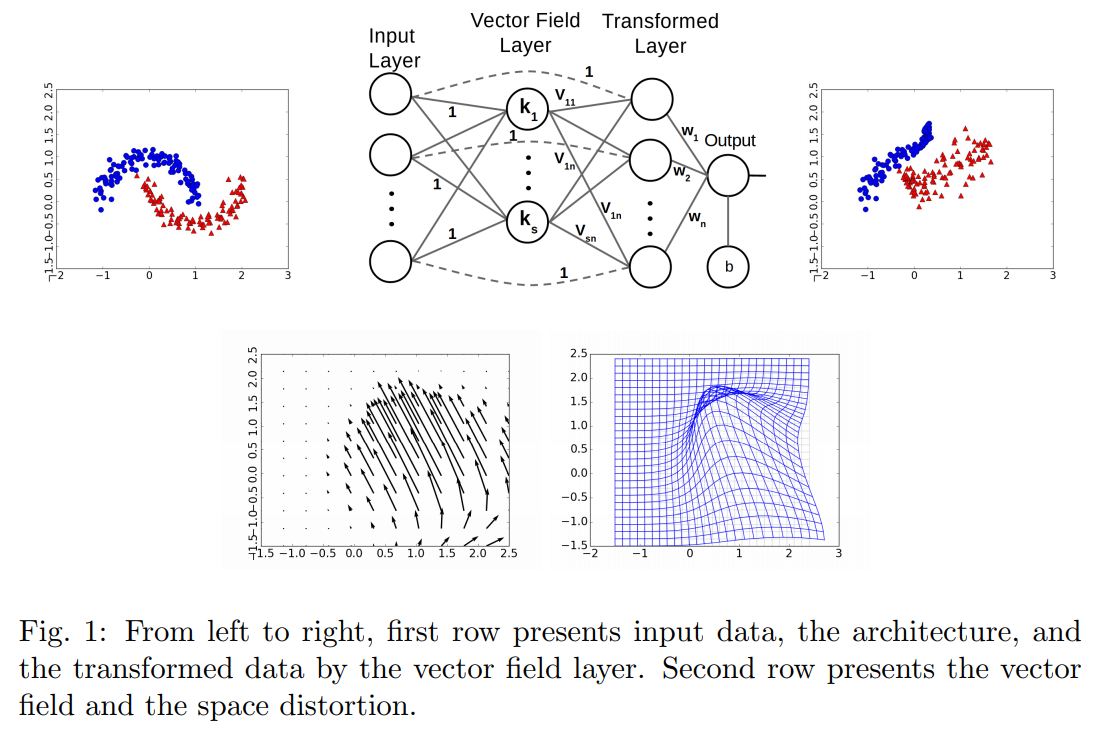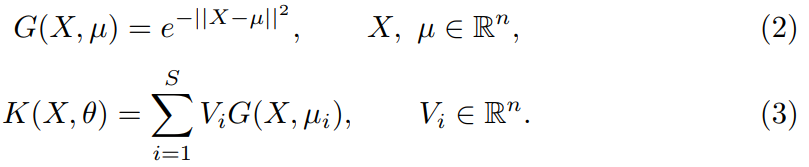The authors propose a novel layer to perform non-linear transformations inside a neural network. They design a Vector Field Layer which learns a vector field to be applied to the input.Let $$X$$ be the input, $$\mu_{i}$$ the means of the $$S$$ gaussians and $$V_{i}$$ the $$S$$ learned vectors.

We can compute $$X_{i+1} = X_{i} + h(K(X_{i}, \theta))$$, the transformed input.

$$h$$ is the step-size, $$\theta$$ are the parameters $$(\mu_{i}, V_{i})$$

$$K(X_{i}, \theta)$$ is a weighted sum of vectors, the weights are a result of a kernel function. (see eq. 2-3).While this is early work, it’s a really interesting method.